# PSEB 12th Class Chemistry Important Questions Chapter 3 Electrochemistry

Punjab State Board PSEB 12th Class Chemistry Important Questions Chapter 3 Electrochemistry Important Questions and Answers.

## PSEB 12th Class Chemistry Important Questions Chapter 3 Electrochemistry

Question 1.
Why on dilution the ∧m of CH3COOH increases drastically while that of CH3COONa increases gradually? [NCERT Exemplar]
In the case of CH3COOH, which is a weak electrolyte, the number of ions increase on dilution due to an increase in degree of dissociation.
CH3COOH + H2o → CH3COO + H3O+

Question 2.
Why is alternating current used for measuring resistance of an electrolytic solution? [NCERT Exemplar]
Alternating current is used to prevent electrolysis so that concentration of ions in the solution remains constant.

Question 3.
Define electrochemical series.
The arrangement of elements in the increasing or decreasing order of their standard reduction potentials is called electrochemical series.Question 4.
What are secondary cells?
Secondary cells are those cells which are rechargeable, i.e., the products can be changed back to reactants.

Question 5.
What is the necessity to use a salt bridge in a Galvanic cell?
To complete the inner circuit and to maintain the electrical neutrality of the electrolytic solutions of the half-cells we use a salt bridge in a Galvanic cell.

Question 6.
Why does a dry cell become dead after a long time even if it has not been used?
Even though not in use, a dry cell becomes dead after some time because the acidic NH4C1 corrodes the zinc container.

Question 7.
What does the negative sign in the expression $$\boldsymbol{E}_{\mathbf{Z n}^{2+} / \mathbf{Z n}}[latex] = -0.76 V mean?[NCERT Exemplar] Answer: It means that Zn is more reactive than hydrogen. When zinc electrode will be connected to SHE, Zn will get oxidised and H+ will get reduced.Question 8. Write the Nemst equation for the cell reaction in the Daniel cell. How will the £cell be affected when concentration of Zn2+ ions is increased? [NCERT Exemplar] Answer: Zn + Cu2+ > Zn2+ + Cu Ecell = [latex]E_{\text {cell }}^{\ominus}$$ – $$\frac{0.059}{2}$$ log $$\frac{\left[\mathrm{Zn}^{2+}\right]}{\left[\mathrm{Cu}^{2+}\right]}$$
Ecellcell decreases when concentration of Zn2+ ions, [Zn2+ ] increases.

Question 9.
What does the negative value of $$E_{\text {cell }}^{\ominus}$$ indicate?
Negative $$E_{\text {cell }}^{\ominus}$$ value means ΔrGΘ will be +ve, and the cell will not work.

Question 10.
Can $$E_{\text {cell }}^{\ominus}$$ or ΔrGΘ for cell reaction ever be equal to zero? [NCERT Exemplar]
No.

Question 1.
State Kohlrausch law of independent migration of ions. Why does the conductivity of a solution decrease with dilution?
Kohlrausch law states that the limiting molar conductivity of an electrolyte can be represented as the sum of the individual contributions of cation and anion of the electrolyte.

In general, if an electrolyte on dissociation gives v+ cations and v anions then its limiting molar conductivity is given by
$$\Lambda_{m}^{\ominus}=v_{+} \lambda_{+}^{0}+v_{-} \lambda_{-}^{0}$$

Where, $$\lambda_{+}^{0}$$ and $$\lambda_{-}^{0}$$ are the limiting molar conductivities of cations and anions respectively.

Conductivity of a solution decreases with dilution. This is due to the fact that the number of ions per unit volume that carry the current in a solution decreases with dilution.Question 2.
Define the following terms:
(i) Fuel cell
(ii) Limiting molar conductivity ()
(i) A fuel cell is a device which converts the energy produced during the combustion of fuels like hydrogen, methanol, methane etc. directly into electrical energy. One of the most successful fuel cell is H2 – O2 fuel cell.

(ii) When concentration approaches zero, the molar conductivity is known
as limiting molar conductivity. It is represented by $$\Lambda_{m}^{\ominus}$$.
$$\Lambda_{m}^{\ominus}$$ (∧b)when → c

Question 3.
(i) Write two advantages of H2 – O2 fuel cell over ordinary cell.
(ii) Equilibrium constant (Kc) for the given cell reaction is 10. Calculate $$\boldsymbol{E}_{\text {cell }}^{\ominus}$$.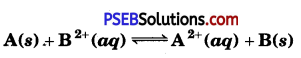Solution:
(i) The two main advantages of H2 – O2 fuel cell over ordinary cell are as follows:

• It has high efficiency of 60%-70%.
• It does not cause any pollution.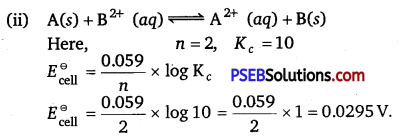Question 4.
Following reactions occur at cathode during the electrolysis of aqueous silver chloride solution:
Ag+(aq) + e → Ag(s) EΘ = + 0.80 V
H+ (aq)+ e → $$\frac{1}{2}$$H2(g) EΘ = 0.00 V
On the basis of their standard reduction electrode potential (EΘ) values, which reaction is feasible at the cathode and why?
The reaction, Ag+ (aq) + e → Ag(s) is feasible at cathode as
cathodic reaction is one which has higher standard reduction electrode potential ($$E_{\text {red }}^{\ominus}$$).

Question 5.
Calculate ∆G and log Kc for the following reaction at 298 K:
2Cr(8) + 3Fe2+(aq) > 2Cr3+ (aq) + 3Fe(s)
Given: $$\boldsymbol{E}_{\text {cell }}^{\ominus}$$ = 0.30 V
Solution: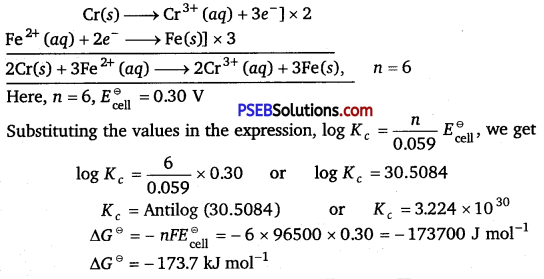Question 6.
Calculate the emf of the following cell at 298 K:
Cr(s)/Cr3+ (0.1M)//Fe<>2+ (0.01M)/(Fe(s) [Given: $$\boldsymbol{E}_{\text {cell }}^{\ominus}$$ = + 0.30 V]
The cell reaction is as follows :
2Cr(s) + 3Fe2+(aq) > 3Fe(s) + 2Cr3+(aq)
For this reaction, n = 6
Now,
Ecell = $$\boldsymbol{E}_{\text {cell }}^{\ominus}$$ – $$\frac{2.303 R T}{n F}$$ log $$\frac{\left[\mathrm{Cr}^{3+}\right]^{2}}{\left[\mathrm{Fe}^{2+}\right]^{3}}$$
Ecell = 0.30 – $$\frac{0.059}{6}$$ log $$\frac{\left[10^{-1}\right]^{2}}{\left[10^{-2}\right]^{3}}$$
Ecell = 0.26VQuestion 7.
The conductivity of 10-3 mol/L acetic acid at 25°C is 4.1 x 10-5 S cm-1. Calculate its degree of dissociation, if $$\Lambda_{m}^{0}$$ for acetic acid at 25°C is 390.5 S cm2 mol-1.
We know that ∧m = $$\frac{1000 \mathrm{~K}}{\mathrm{C}}$$
m = $$\frac{1000 \times 4.1 \times 10^{-5}}{10^{-3}}$$
= 41 S cm2 mol-1
Now, α = $$\frac{\Lambda_{m}^{c}}{\Lambda_{m}^{0}}$$
= $$\frac{41}{390.5}$$ = 0.105 390.5

Question 8.
(i) Solutions of two electrolytes ‘A’ and ‘B’ are diluted. The limiting molar conductivity of ‘B’ increases 1.5 times while that of ‘A’ increases 25 times. Which of the two is a strong electrolyte? Justify your answer.
(ii) The products of electrolysis of aqueous NaCl at the respective electrodes are:
Cathode: H2
Anode: Cl2 and not 02. Explain.
(i) ‘B’ is a strong electrolyte.
Because a strong electrolyte is already dissociated into ions, but on dilution inter ionic forces are overcome, ions are free to move. So, there is slight increase in molar conductivity on dilution.

(ii) On anode water should get oxidised in preference to Cl but due to overvoltage/over potential Cl” is oxidised in preference to water.Question 1.
(a) The conductivity of 0.20 mol L-1 solution of KC1 is 2.48 x 10-2 S cm-1. Calculate its molar conductivity and degree of dissociation (α). Given λ0 (K+) = 73.5 S cm2 mol-1 and λ0 (Cl) = 76.5 S cm2 mol-1.

(b) What type of battery is mercury cell? Why is it more advantageous than dry cell?
(a) Substituting K =2.48 x 10-2 S cm-1, M = 0.20 molL-1 in the expression ∧m = $$\frac{K \times 1000}{M}[latex] , we get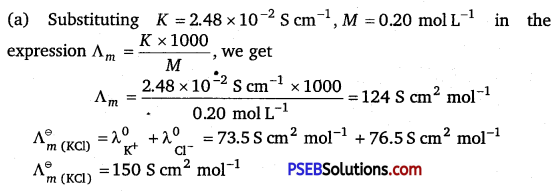Substituting ∧m = 124 S cm2 mol-1, [latex]\Lambda_{m}^{\ominus}$$ = 150 S cm2 mol-1 in the expression α = $$\frac{\Lambda_{m}}{\Lambda_{m}^{\ominus}}$$, we get
Degree of dissociation, α = $$\frac{124 \mathrm{~s} \mathrm{~cm}^{2} \mathrm{~mol}^{-1}}{150 \mathrm{~s} \mathrm{~cm}^{2} \mathrm{~mol}^{-1}}$$ = 82666
α = 82.67%

(b) Primary cell. Mercury cell is more advantageous than dry cell because its cell potential remains constant during its life as the overall reaction does not involve any ion in solution whose concentration can change during its life period.

Question 2.
(i) The conductivity of 0.001 mol L-1 solution of CH3COOH is 3.905 x 10-5 S cm-1. Calculate its molar conductivity and degree of dissociation (α).
Given λ0 (H+) = 349.6 S cm2 mol-1 and λ0 (CH3COO ) = 40.9 S cm2 mol-1

(ii) Define electrochemical cell. What happens if external potential applied becomes greater than $$\boldsymbol{E}_{\text {cell }}^{\ominus}$$ of electrochemical cell?
Solution: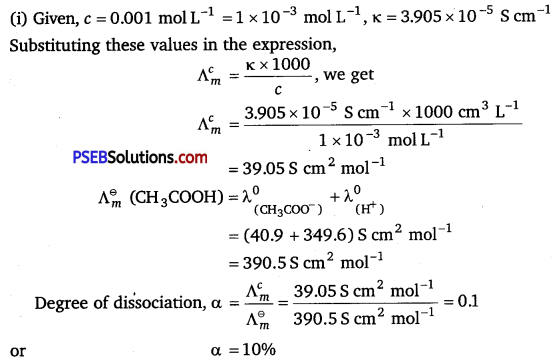(b) A device which is used to convert chemical energy produced in a redox reaction into electrical energy is called an electrochemical cell.
If external potential applied becomes greater than $$\boldsymbol{E}_{\text {cell }}^{\ominus}$$ of
electrochemical cell, the reaction gets reversed and the electrochemical cell function as an electrolytic cell.Question 3.
Calculate e.m.f and ΔG for the following cell at 298 K:
Mg(s) | Mg2+ (0.01 M) || Ag+ (0.0001 M) | Ag(s)
Given: $$\underset{\left(\mathrm{Mg}^{2+} / \mathrm{Mg}\right)}{\boldsymbol{E}^{\circ}}$$ = -2.37V, $$\boldsymbol{E}^{\ominus}{\left(\mathbf{A g}^{+} / \mathbf{A g}\right)}$$ = + 0.80V
Solution: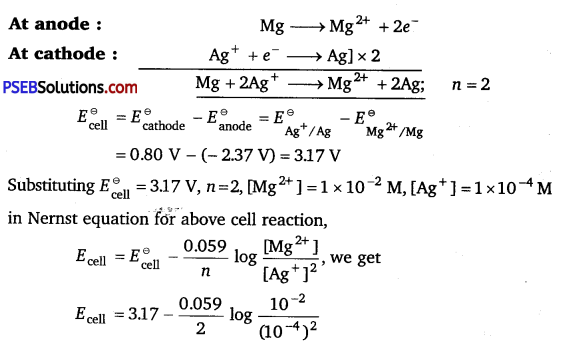Ecell = 3.17 – 0.0295 log 106
Ecell = 3.17 – 0.177 V = 2.993 V
Ecell = 2.993 V
Substituting n = 2, F = 96500 C mol-1, Ecell = 2.993 V in the
expression, ΔG = – nFEcell, we get
ΔG = nFEcell = -2 x 96500 C mol-1 x 2.993V
ΔG = – 577649 J mol-1 = – 577.649 kJ mol-1